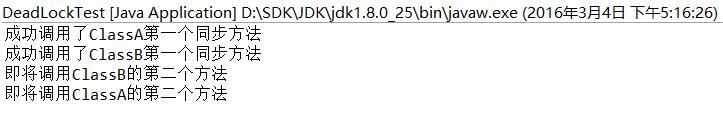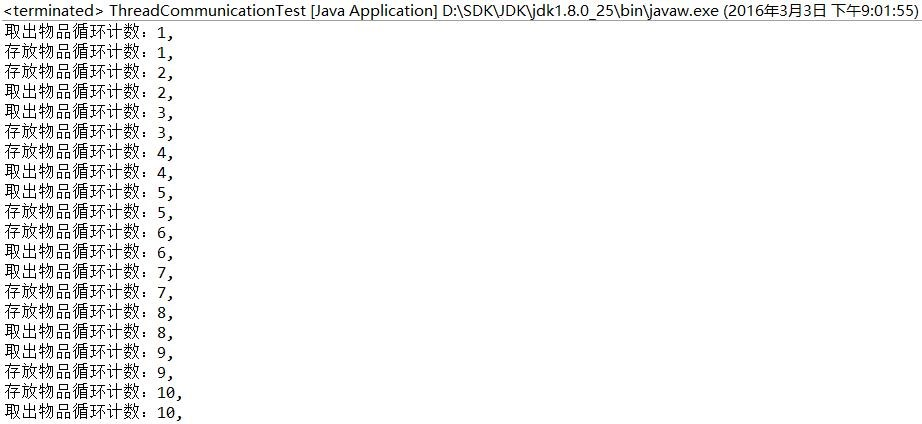jisonami A Java Dev Engineer

# Java多线程（三）--线程锁

2016-09-05

#### 使用synchronized关键字进行加锁

``````synchronized (obj) {
// 在大括号内编写的代码就叫同步代码块
}
``````

``````public class SynchronizedBlockTest {
public Double a;
//synchronized (a) {
a = a + b;
b = a + b;
a = a + b;
//}
}
}
``````

``````public class SynchronizedMethodTest {
public int sum;
sum = sum + aValue;
}
public synchronized void sub(int aValue){
sum = sum - aValue;
}
}
``````

``````public class SynchronizedMethodTest {
public static int sum;
public static synchronized void add(int aValue){
sum = sum + aValue;
}
public static synchronized void sub(int aValue){
sum = sum - aValue;
}
}
````````````class X {
private final ReentrantLock lock = new ReentrantLock();
public void m() {
lock.lock();
try {
// 这里是需要加同步锁的代码
} finally {
lock.unlock()
}
}
}
``````

``````public class X {
try {
// 需要加读取锁的代码
}
finally {
}
}
public void writeLockTest(){
rwLock.writeLock().lock();
try {
// 需要加写入锁的代码
}
finally {
rwLock.writeLock().unlock();
}
}
}
``````

#### 使用特殊域变量（volatile）实现线程同步

volatile关键字为域变量的访问提供了一种免锁机制，使用volatile修饰域相当于告诉虚拟机该域可能会被其他线程更新，因此每次使用该域就要重新计算，而不是使用寄存器中的值，volatile不会提供任何原子操作，它也不能用来修饰final类型的变量。

``````public class VolatileTest {
public volatile AtomicInteger sum = new AtomicInteger(0);
}
public void sub(int aValue){
}
}
``````

#### 程序出现死锁

``````public class DeadLockTest{
final static ClassA a = new ClassA();
final static ClassB b = new ClassB();
public static void main(String[] args) {
// 此处使用了Java8的lambda表达式简化线程代码
new Thread( () -> a.firstMethod(b) ).start();
new Thread( () -> b.firstMethod(a) ).start();
}
}
class ClassA {
public synchronized void firstMethod(ClassB b){
System.out.println("成功调用了ClassA第一个同步方法");
try {
} catch (InterruptedException e) {
e.printStackTrace();
}
System.out.println("即将调用ClassB的第二个方法");
b.secondMethod();
}
public synchronized void secondMethod(){
System.out.println("成功调用了ClassA第二个同步方法");
}
}
class ClassB {
public synchronized void firstMethod(ClassA a){
System.out.println("成功调用了ClassB第一个同步方法");
try {
} catch (InterruptedException e) {
e.printStackTrace();
}
System.out.println("即将调用ClassA的第二个方法");
a.secondMethod();
}
public synchronized void secondMethod(){
System.out.println("成功调用了ClassB第二个同步方法");
}
}
``````### 线程通信

#### 使用Object类的wait()、notify()和notifyAll()方法

wait()方法有三个重载的形式，Java6的文档上是这么写的：

wait()方法，在其他线程调用此对象的 notify() 方法或 notifyAll() 方法前，导致当前线程等待。

wait(long timeout)方法，在其他线程调用此对象的 notify() 方法或 notifyAll() 方法，或者超过指定的时间量前，导致当前线程等待。

wait(long timeout, int nanos)方法，在其他线程调用此对象的 notify() 方法或 notifyAll() 方法，或者其他某个线程中断当前线程，或者已超过某个实际时间量前，导致当前线程等待。

``````public class ThreadCommunicationTest{
private boolean depositFlag = false;
public synchronized void deposit(){
if(depositFlag){
try {
wait();
} catch (InterruptedException e) {
e.printStackTrace();
}
} else {
depositFlag = true;
notifyAll();
}
}
public synchronized void take(){
if(!depositFlag){
try {
wait();
} catch (InterruptedException e) {
e.printStackTrace();
}
} else {
depositFlag = false;
notifyAll();
}
}
public static void main(String[] args) {
}
}
this.tct = tct;
}
@Override
public void run() {
for (int i = 0; i < 10; i++) {
System.out.println("存放物品循环计数："+(i+1)+",");
tct.deposit();
}
}
}
this.tct = tct;
}
@Override
public void run() {
for (int i = 0; i < 10; i++) {
System.out.println("取出物品循环计数："+(i+1)+",");
tct.take();
}
}
}
``````#### 使用Condition实现线程通信

Lock接口声明了一个newCondition()方法，这个方法可以用来获得一个Condition对象，这个对象就相当于使用synchronized加锁时的同步监视器，里面有三个类似wait()、notify()和notifyAll()的方法，分别是await()、signal()和signalAll()方法，功能也是一样的。

await()方法，造成当前线程在接到信号或被中断之前一直处于等待状态。

signal()方法，唤醒一个等待线程。

signalAll()方法， 唤醒所有等待线程。

``````public class ThreadCommunicationTest{
private boolean depositFlag = false;
private final ReentrantLock lock = new ReentrantLock();
private final Condition condition = lock.newCondition();
public void deposit(){
lock.lock();
try {
if(depositFlag){
try {
condition.await();
} catch (InterruptedException e) {
e.printStackTrace();
}
} else {
depositFlag = true;
condition.signalAll();
}
}
finally {
lock.unlock();
}
}
public void take(){
lock.lock();
try {
if(!depositFlag){
try {
condition.await();
} catch (InterruptedException e) {
e.printStackTrace();
}
} else {
depositFlag = false;
condition.signalAll();
}
}
finally {
lock.unlock();
}
}
public static void main(String[] args) {